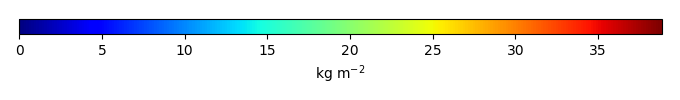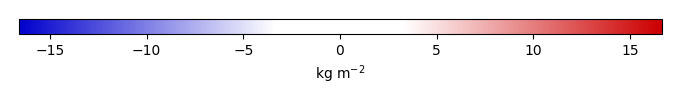# Mean State

Period Mean (original grids) [Pg]
Model Period Mean (intersection) [Pg]
Model Period Mean (complement) [Pg]
Benchmark Period Mean (intersection) [Pg]
Benchmark Period Mean (complement) [Pg]
Bias [kg m-2]
Bias Score 
Spatial Distribution Score 
Overall Score 
Benchmark [-] 30.1
CLM4 [-] 582. 22.9 559. 29.9 0.195 -0.538 0.615 0.774 0.694
CLM4.5 [-] 560. 27.8 532. 29.9 0.195 0.0652 0.600 0.827 0.714
CLM5 [-] 589. 29.0 560. 29.9 0.195 0.319 0.597 0.839 0.718
Period Mean (original grids) [Pg]
Model Period Mean (intersection) [Pg]
Model Period Mean (complement) [Pg]
Benchmark Period Mean (intersection) [Pg]
Benchmark Period Mean (complement) [Pg]
Bias [kg m-2]
Bias Score 
Spatial Distribution Score 
Overall Score 
Benchmark [-] 1.44
CLM4 [-] 4.27 0.825 3.37 1.41 0.0279 -0.354 0.498 0.646 0.572
CLM4.5 [-] 9.20 3.15 5.91 1.41 0.0279 1.69 0.483 0.805 0.644
CLM5 [-] 16.4 5.84 10.4 1.41 0.0279 4.22 0.263 0.351 0.307
Period Mean (original grids) [Pg]
Model Period Mean (intersection) [Pg]
Model Period Mean (complement) [Pg]
Benchmark Period Mean (intersection) [Pg]
Benchmark Period Mean (complement) [Pg]
Bias [kg m-2]
Bias Score 
Spatial Distribution Score 
Overall Score 
Benchmark [-] 0.00649
CLM4 [-] 5.39 0.00905 5.08 0.00649 0.00778 0.433 0.0945 0.264
CLM4.5 [-] 11.4 0.0173 11.1 0.00649 0.148 0.562 0.793 0.677
CLM5 [-] 11.9 0.0238 11.6 0.00649 0.192 0.544 0.674 0.609
Period Mean (original grids) [Pg]
Model Period Mean (intersection) [Pg]
Model Period Mean (complement) [Pg]
Benchmark Period Mean (intersection) [Pg]
Benchmark Period Mean (complement) [Pg]
Bias [kg m-2]
Bias Score 
Spatial Distribution Score 
Overall Score 
Benchmark [-] 17.4
CLM4 [-] 16.4 16.0 0.277 17.3 0.0180 -0.0107 0.674 0.911 0.792
CLM4.5 [-] 20.4 19.9 0.343 17.3 0.0180 1.01 0.679 0.915 0.797
CLM5 [-] 17.8 17.3 0.314 17.3 0.0180 0.419 0.686 0.919 0.803
Period Mean (original grids) [Pg]
Model Period Mean (intersection) [Pg]
Model Period Mean (complement) [Pg]
Benchmark Period Mean (intersection) [Pg]
Benchmark Period Mean (complement) [Pg]
Bias [kg m-2]
Bias Score 
Spatial Distribution Score 
Overall Score 
Benchmark [-] 1.52
CLM4 [-] 25.1 1.02 24.2 1.46 0.0621 -0.890 0.476 0.682 0.579
CLM4.5 [-] 34.8 1.47 33.5 1.46 0.0621 1.42 0.601 0.759 0.680
CLM5 [-] 56.8 2.46 54.5 1.46 0.0621 7.01 0.557 0.882 0.720
Period Mean (original grids) [Pg]
Model Period Mean (intersection) [Pg]
Model Period Mean (complement) [Pg]
Benchmark Period Mean (intersection) [Pg]
Benchmark Period Mean (complement) [Pg]
Bias [kg m-2]
Bias Score 
Spatial Distribution Score 
Overall Score 
Benchmark [-] 9.78
CLM4 [-] 5.11 4.98 0.169 9.71 0.0683 -1.19 0.547 0.421 0.484
CLM4.5 [-] 3.42 3.26 0.205 9.71 0.0683 -1.67 0.475 0.261 0.368
CLM5 [-] 3.49 3.35 0.157 9.71 0.0683 -1.61 0.492 0.568 0.530

# Temporally integrated period mean

BENCHMARK MEANMODEL MEANBIASBIAS SCORESPATIAL TAYLOR DIAGRAMMODEL COLORS# Spatially integrated regional mean

MODEL COLORS# All Models

BenchmarkCLM4CLM4.5CLM5